Next: Magnetic energy Up: Magnetic induction Previous: Self-inductance

## Mutual inductance

Consider, now, two long thin solenoids, one wound on top of the other. The length of each solenoid is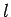, and the common radius is. Suppose that the bottom coil has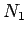turns per unit length, and carries a current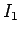. The magnetic flux passing through each turn of the top coil is, and the total flux linking the top coil is therefore, whereis the number of turns per unit length in the top coil. It follows that the mutual inductance of the two coils, defined, is given by(916)

Recall that the self-inductance of the bottom coil is(917)

and that of the top coil is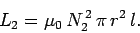(918)

Hence, the mutual inductance can be written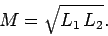(919)

Note that this result depends on the assumption that all of the flux produced by one coil passes through the other coil. In reality, some of the flux leaks out, so that the mutual inductance is somewhat less than that given in the above formula. We can write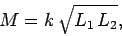(920)

where the constantis called the coefficient of coupling, and lies in the range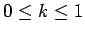.

Suppose that the two coils have resistances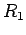and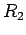. If the bottom coil has an instantaneous currentflowing through it, and a total voltage drop, then the voltage drop due to its resistance is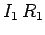. The voltage drop due to the back e.m.f. generated by the self-inductance of the coil is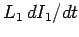. There is also a back e.m.f. due to inductive coupling with the top coil. We know that the flux through the bottom coil due to the instantaneous currentflowing in the top coil is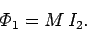(921)

Thus, by Faraday's law and Lenz's law, the e.m.f. induced in the bottom coil is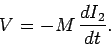(922)

The voltage drop across the bottom coil due to its mutual inductance with the top coil is minus this expression. Thus, the circuit equation for the bottom coil is(923)

Likewise, the circuit equation for the top coil is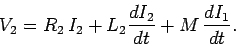(924)

Here,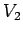is the total voltage drop across the top coil.

Suppose that we suddenly connect a battery of e.m.f.to the bottom coil, at time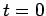. The top coil is assumed to be open-circuited, or connected to a voltmeter of very high internal resistance, so that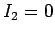. What is the e.m.f. generated in the top coil? Since, the circuit equation for the bottom coil is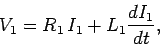(925)

whereis constant, and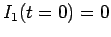. We have already seen the solution to this equation:(926)

The circuit equation for the top coil is(927)

giving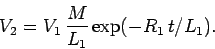(928)

It follows from Eq. (920) that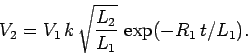(929)

Since, we obtain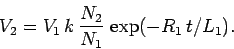(930)

Note thatis discontinuous at. This is not a problem, since the resistance of the top circuit is infinite, so there is no discontinuity in the current (and, hence, in the magnetic field). But, what about the displacement current, which is proportional to? Surely, this is discontinuous at(which is clearly unphysical)? The crucial point, here, is that we have specifically neglected the displacement current in all of our previous analysis, so it does not make much sense to start worrying about it now. If we had retained the displacement current in our calculations, then we would have found that the voltage in the top circuit jumps up, at, on a time-scale similar to the light traverse time across the circuit (i.e., the jump is instantaneous to all intents and purposes, but the displacement current remains finite).

Now,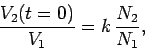(931)

so if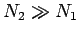then the voltage in the bottom circuit is considerably amplified in the top circuit. This effect is the basis for old-fashioned car ignition systems. A large voltage spike is induced in a secondary circuit (connected to a coil with very many turns) whenever the current in a primary circuit (connected to a coil with not so many turns) is either switched on or off. The primary circuit is connected to the car battery (whose e.m.f. is typically 12 volts). The switching is done by a set of points, which are mechanically opened and closed as the engine turns. The large voltage spike induced in the secondary circuit, as the points are either opened or closed, causes a spark to jump across a gap in this circuit. This spark ignites a petrol/air mixture in one of the cylinders. We might think that the optimum configuration is to have only one turn in the primary circuit, and lots of turns in the secondary circuit, so that the ratio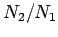is made as large as possible. However, this is not the case. Most of the magnetic field lines generated by a single turn primary coil are likely to miss the secondary coil altogether. This means that the coefficient of couplingis small, which reduces the voltage induced in the secondary circuit. Thus, we need a reasonable number of turns in the primary coil in order to localize the induced magnetic field, so that it links effectively with the secondary coil.Next: Magnetic energy Up: Magnetic induction Previous: Self-inductance
Richard Fitzpatrick 2006-02-02